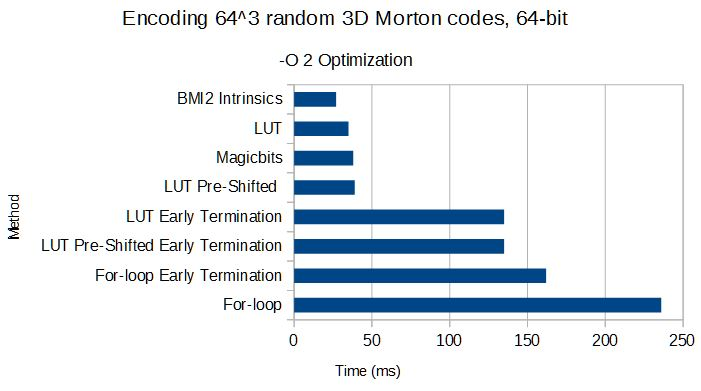# Using the BMI2 instruction set to encode / decode Morton Codes

I’ve updated my Libmorton library to use BMI2 CPU instructions if they are available. You can read more about Morton codes, their uses and the library in the previous blogposts about the subject.

As a quick recap, the problem is that you easily want to interleave bits from a set of coordinates x, y and z (in the 3D case) to form a Morton code, which assigns each coordinate a position on the Z-order curve, (also known as the Lebesgue curve) and the other way around. Applications of Morton codes can be found in rendering large datasets, databases, etc….

Here’s a simple example:

• (x,y,z) = (5,9,1) = (0101,1001,0001)
• Interleaving the bits results in: 010001000111 = 1095 th cell along the Z-curve.

Although the operation is easy to do visually, it is somewhat tricky to implement efficiently, which is why I started Libmorton as a fun little project to collect and compare all of the different possibilities, and benchmark the different implementations.

On newer Intel-based (Haswell and up) and AMD processors, the Bit Manipulation Instruction Set (or BMI) has been available for a while. The second iteration of this instruction set (BMI2) has some interesting functions we can leverage to do the extracting and depositing of bits using only a few clock cycles: Parallel Bit Deposit (`PDEP`) and Parallel Bit Extract (`PEXT`). Both of these functions take a source input and a mask as parameters, and return a low-bit-starting listing of the mask-selected bits.

The implementation for encoding and decoding looks as follows:

[code language=”cpp”]

template<typename morton, typename coord>
inline morton encode(const coord x, const coord y, const coord z){
morton m = 0;
m |= pdep(static_cast<morton>(x), static_cast<morton>(BMI_X_MASK))
return m;
}

template<typename morton, typename coord>
inline void decode(const morton m, coord& x, coord& y, coord& z){
x = static_cast<coord>(pext(m, static_cast<morton>(BMI_X_MASK)));
y = static_cast<coord>(pext(m, static_cast<morton>(BMI_Y_MASK)));
z = static_cast<coord>(pext(m, static_cast<morton>(BMI_Z_MASK)));
}
[/code]

As a quick test, I ran a few quick tests using all the 3D encoding/decoding methods present in the library at this point. Testing on 128³ Morton codes (yes, I know the graph title says 64³) from randomly generated coordinates in the full 3D space, in 64-bit mode, with -O 2 optimization. Compiler is MSVC 14.0 on an Intel I7 5500U CPU (a Broadwell laptop CPU).

As a quick recap, these are the different methods tested:

• For loop and For loop with Early termination: A simple, “dumb”, for-loop based method for encoding, which loops over every input coordinate bit and deposits it in the final Morton code. The Early Termination version checks for the highest bit set and stops early.
• Magicbits: A method which uses special bitmasks and shifts to separate the bits from the coordinates into the final Morton code
• Lookup Table (LUT): Methods that use a pre-computed table which already contains the correct Morton code for 8 bits of the input.
• Pre-shifted: 2 extra tables which already shift the input for y and z coordinates as well
• Early Termination: Method which checks for highest set bit in the input and tries to stop early.A few things are clear:

• None of the Early Termination approaches are worth the work, instead in the case of the dumb For-loop, where extra loops of shifting useless zeroes around is very expensive.
• The LUT-based approaches and Magicbits method are comparable in speed, with pre-shifting not making a lot of difference, and probably not worth it considering the impact on caching when the LUT tables grow in size.
• As expected, the BMI2 intrinsics are exactly the operations that are needed to implement Morton encoding efficiently.

More results follow for 32-bit and decoding scenarios, I expect the same conclusions.

To use this new method, make sure that __BMI2__ is defined when you’re compiling your project. GCC should automatically set that, but MSVC sometimes needs a hint.

This site uses Akismet to reduce spam. Learn how your comment data is processed.